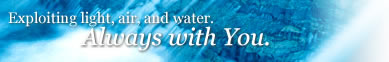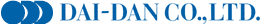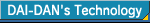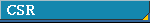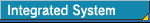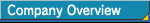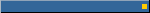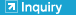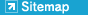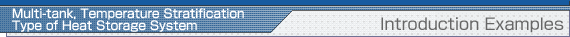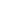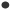OverviewIntroduction ConditionsIntroduction ExamplesFrequently Asked QuestionsIntroduction AccomplishmentsMulti-tank, Temperature Stratification Type of Heat Storage System: Introduction ExamplesA certain commercial facility (department store), which has a total water amount of 2,510 m3 (approx. 3-m water depth), and of two-tank parallel-connected type having a total of 28 tanks. Through the conversion to temperature stratification system, the efficiency of the heat storage tank was improved to approximately 90% and further the quantity of heat possible to be stored was multiplied by a factor of approximately 1.5 times. (With mixed tank systems, the efficiency is only 50 to 60%.) In addition, to minimize the piping costs, giving considerations to piping resistance and flow velocity, we at DAI-DAN performed trial calculation of distribution flow rate, thus providing a piping layout shown in figure below. (The thermocouple is attached for the measurement, which can be omitted.) Furthermore, even if the water depth is approximately 1.8 m, i.e., the same level as that of ordinary underground slab, the temperature stratification of heat storage tanks will be able to be installed. (The efficiency of heat storage tanks slightly drops to approximately 80%.) Click here to enlarge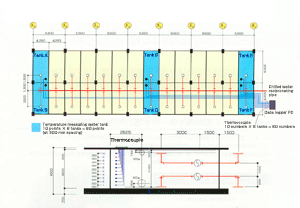Copyright 2004 DAI-DAN Co.,Ltd.